# 1.3c: Adding topography to geological models¶

import gempy as gp
import numpy as np
import matplotlib.pyplot as plt
import os


## 1. The common procedure to set up a model:¶

data_path = 'https://raw.githubusercontent.com/cgre-aachen/gempy_data/master/'

geo_model = gp.create_model('Single_layer_topo')
gp.init_data(geo_model, extent=[450000, 460000, 70000, 80000, -1000, 500],
resolution=[50, 50, 50],
path_i=data_path + "/data/input_data/tut-ch1-7/onelayer_interfaces.csv",
path_o=data_path + "/data/input_data/tut-ch1-7/onelayer_orient.csv")


Out:

Active grids: ['regular']

Single_layer_topo  2020-10-27 13:19


use happy spring colors!

geo_model.surfaces.colors.change_colors({'layer1': '#ff8000', 'basement': '#88cc60'})

gp.map_stack_to_surfaces(geo_model, {'series': ('layer1', 'basement')})

surface series order_surfaces color id
0 layer1 series 1 #ff8000 1
1 basement series 2 #88cc60 2

s = {'s1': ([450000, 75000], [460000, 75500], [100, 100])}
geo_model.set_section_grid(s)


Out:

Active grids: ['regular' 'sections']

start stop resolution dist
s1 [450000, 75000] [460000, 75500] [100, 100] 10012.49

### 2 a. Load from raster file¶

This is to make it work in sphinx gallery

cwd = os.getcwd()
if not 'examples' in cwd:
path_dir = os.getcwd() + '/examples/tutorials/ch5_probabilistic_modeling'
else:
path_dir = cwd

fp = path_dir + "/../../data/input_data/tut-ch1-7/bogota.tif"

geo_model.set_topography(source='gdal', filepath=fp)
gp.plot_2d(geo_model, show_topography=True, section_names=['topography'], show_lith=False,
show_boundaries=False,
kwargs_topography={'cmap': 'gray', 'norm': None}
)
plt.show()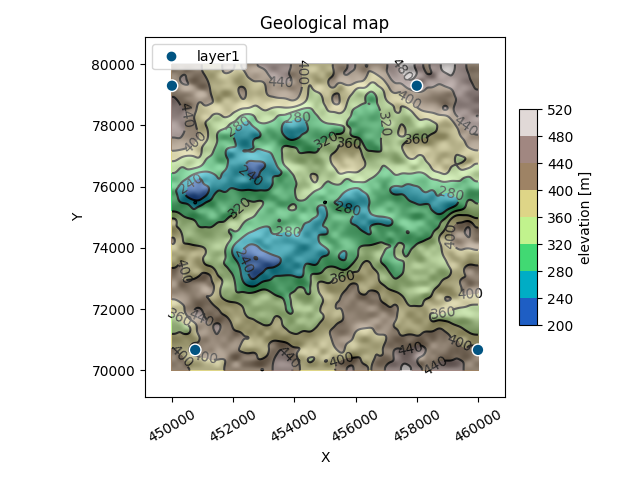Out:

Cropped raster to geo_model.grid.extent.
depending on the size of the raster, this can take a while...
storing converted file...
Active grids: ['regular' 'topography' 'sections']


### 2 b. create fun topography¶

If there is no topography file, but you think that your model with topography would look significantly cooler, you can use gempys set_topography function to generate a random topography based on a fractal grid:

sphinx_gallery_thumbnail_number = 2

geo_model.set_topography(source='random')
gp.plot_2d(geo_model, show_topography=True, section_names=['topography'])
plt.show()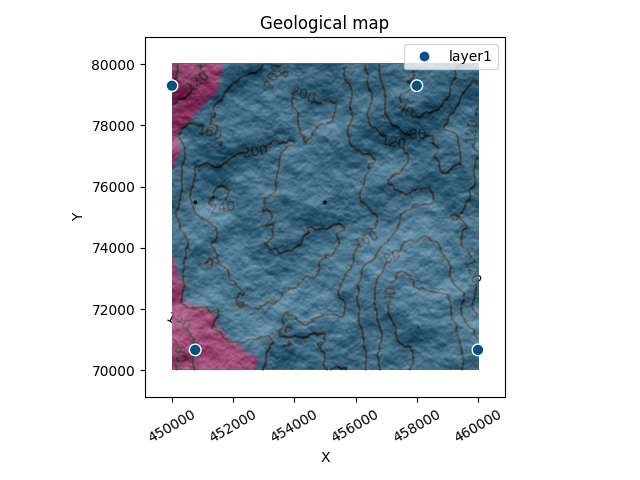Out:

[200. 500.]
Active grids: ['regular' 'topography' 'sections']


It has additional keywords to play around with:

• fd: fractal dimension:

defaults to 2.0. The higher (try 2.9), the rougher the landscape will be.

• d_z: height difference:

If none, last 20% of the model in z direction.

• extent:

extent in xy direction. If none, geo_model.grid.extent is used.

• resolution:

resolution of the topography array. If none, geo_model.grid.resoution is used. Increasing the resolution leads to much nicer geological maps!

geo_model.set_topography(source='random', fd=1.9, d_z=np.array([0, 250]),
resolution=np.array([200, 200]))


Out:

Active grids: ['regular' 'topography' 'sections']

Grid Object. Values:
array([[450100.        ,  70100.        ,   -985.        ],
[450100.        ,  70100.        ,   -955.        ],
[450100.        ,  70100.        ,   -925.        ],
...,
[460000.        ,  75500.        ,    469.6969697 ],
[460000.        ,  75500.        ,    484.84848485],
[460000.        ,  75500.        ,    500.        ]])


Note that each time this function is called, a new random topography is created. If you particularly like the generated topography or if you have loaded a large file with gdal, you can save the topography object and load it again later:

save:

geo_model._grid.topography.save('test_topo')


geo_model.set_topography(source='saved', filepath='test_topo.npy')


Out:

Active grids: ['regular' 'topography' 'sections']

Grid Object. Values:
array([[450100.        ,  70100.        ,   -985.        ],
[450100.        ,  70100.        ,   -955.        ],
[450100.        ,  70100.        ,   -925.        ],
...,
[460000.        ,  75500.        ,    469.6969697 ],
[460000.        ,  75500.        ,    484.84848485],
[460000.        ,  75500.        ,    500.        ]])


## Compute model¶

gp.set_interpolator(geo_model)


Out:

Setting kriging parameters to their default values.
Compiling theano function...
Level of Optimization:  fast_compile
Device:  cpu
Precision:  float64
Number of faults:  0
Compilation Done!
Kriging values:
values
range            1.4e+04
$C_o$            4.8e+06
drift equations      

<gempy.core.interpolator.InterpolatorModel object at 0x7ff9ba1bf910>

gp.compute_model(geo_model, compute_mesh=False, set_solutions=True)


Out:

Lithology ids
[2. 2. 2. ... 1. 1. 1.]


### Visualize:¶

Now, the solutions object does also contain the computed geological map. It can be visualized using the 2D and 3D plotting functionality:

gp.plot_2d(geo_model, show_topography=True, section_names=['topography'], show_boundaries=False, show_data=True)
plt.show()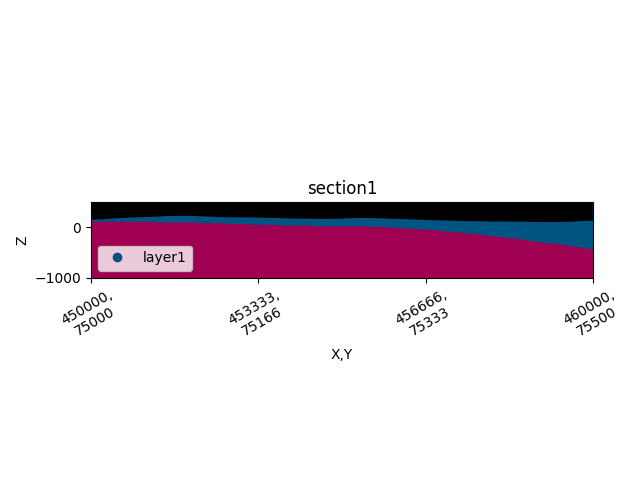gp.plot_2d(geo_model, show_topography=True, section_names=['s1'])
plt.show()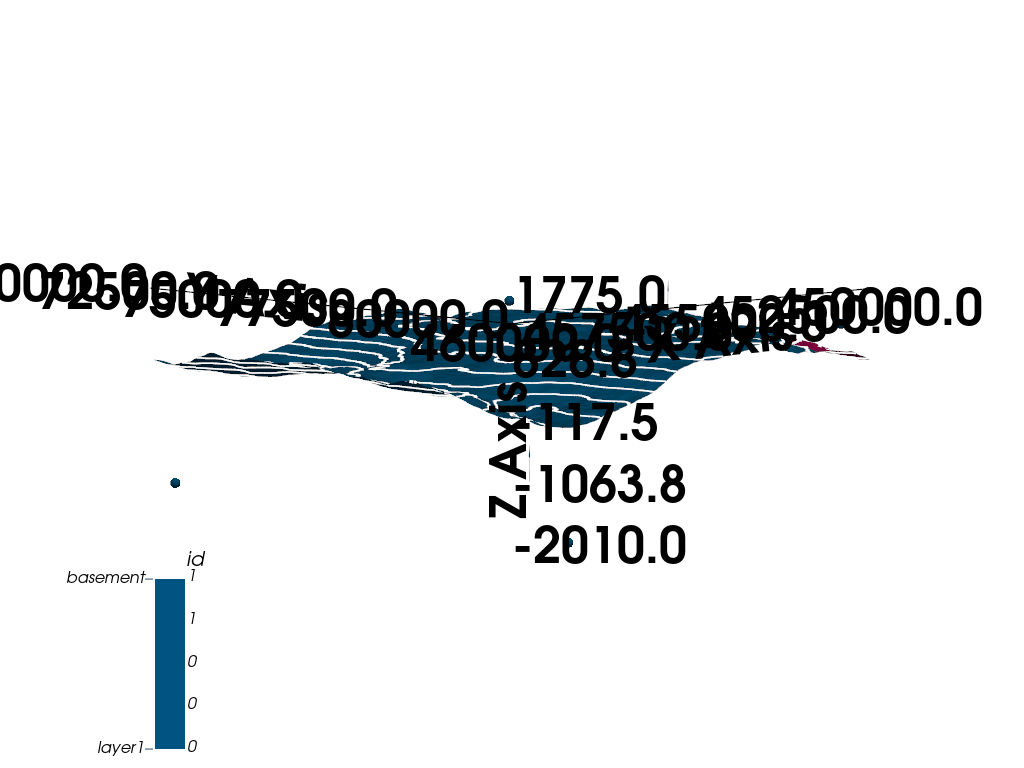g3d = gp.plot_3d(geo_model,
show_topography=True,
show_lith=False,
show_surfaces=False,
show_results=False,
ve=5)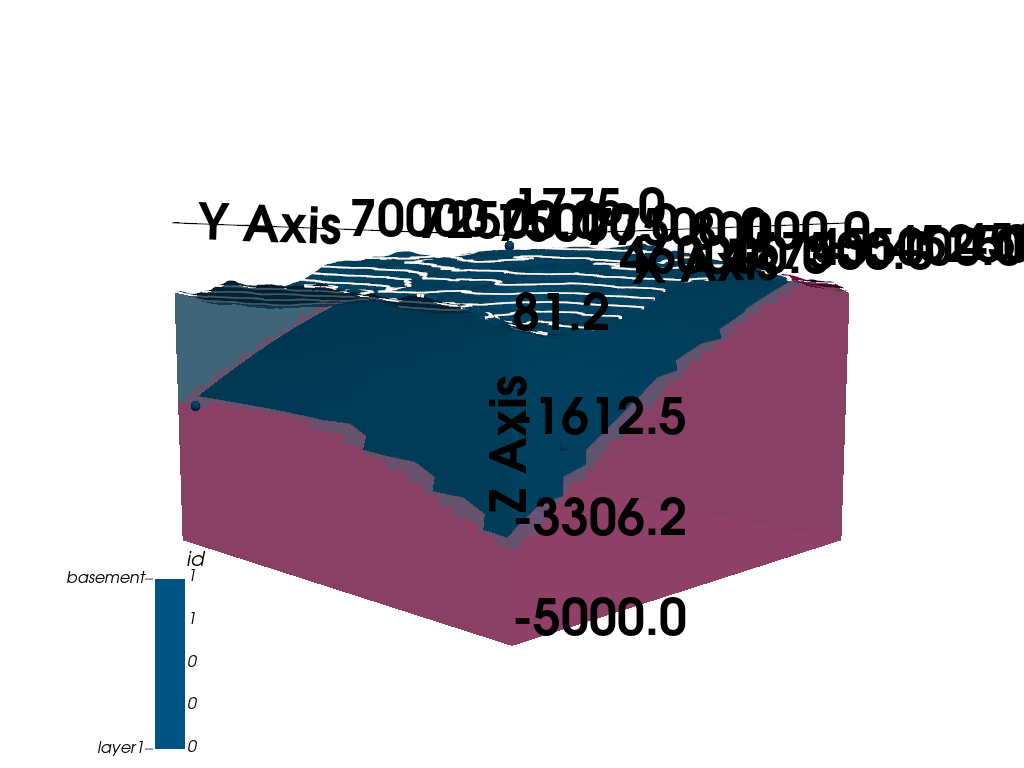sphinx_gallery_thumbnail_number = 3

g3d = gp.plot_3d(geo_model,
show_topography=True,
show_lith=True,
show_surfaces=True,
ve=5)Total running time of the script: ( 0 minutes 20.766 seconds)

Gallery generated by Sphinx-Gallery